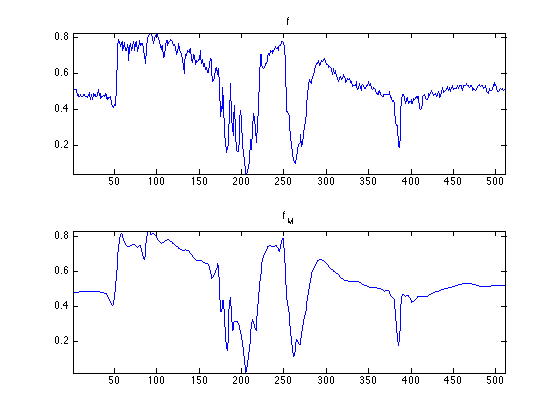$\newcommand{\NN}{\mathbb{N}} \newcommand{\CC}{\mathbb{C}} \newcommand{\GG}{\mathbb{G}} \newcommand{\LL}{\mathbb{L}} \newcommand{\PP}{\mathbb{P}} \newcommand{\QQ}{\mathbb{Q}} \newcommand{\RR}{\mathbb{R}} \newcommand{\VV}{\mathbb{V}} \newcommand{\ZZ}{\mathbb{Z}} \newcommand{\FF}{\mathbb{F}} \newcommand{\KK}{\mathbb{K}} \newcommand{\UU}{\mathbb{U}} \newcommand{\EE}{\mathbb{E}} \newcommand{\Aa}{\mathcal{A}} \newcommand{\Bb}{\mathcal{B}} \newcommand{\Cc}{\mathcal{C}} \newcommand{\Dd}{\mathcal{D}} \newcommand{\Ee}{\mathcal{E}} \newcommand{\Ff}{\mathcal{F}} \newcommand{\Gg}{\mathcal{G}} \newcommand{\Hh}{\mathcal{H}} \newcommand{\Ii}{\mathcal{I}} \newcommand{\Jj}{\mathcal{J}} \newcommand{\Kk}{\mathcal{K}} \newcommand{\Ll}{\mathcal{L}} \newcommand{\Mm}{\mathcal{M}} \newcommand{\Nn}{\mathcal{N}} \newcommand{\Oo}{\mathcal{O}} \newcommand{\Pp}{\mathcal{P}} \newcommand{\Qq}{\mathcal{Q}} \newcommand{\Rr}{\mathcal{R}} \newcommand{\Ss}{\mathcal{S}} \newcommand{\Tt}{\mathcal{T}} \newcommand{\Uu}{\mathcal{U}} \newcommand{\Vv}{\mathcal{V}} \newcommand{\Ww}{\mathcal{W}} \newcommand{\Xx}{\mathcal{X}} \newcommand{\Yy}{\mathcal{Y}} \newcommand{\Zz}{\mathcal{Z}} \newcommand{\al}{\alpha} \newcommand{\la}{\lambda} \newcommand{\ga}{\gamma} \newcommand{\Ga}{\Gamma} \newcommand{\La}{\Lambda} \newcommand{\Si}{\Sigma} \newcommand{\si}{\sigma} \newcommand{\be}{\beta} \newcommand{\de}{\delta} \newcommand{\De}{\Delta} \renewcommand{\phi}{\varphi} \renewcommand{\th}{\theta} \newcommand{\om}{\omega} \newcommand{\Om}{\Omega} \renewcommand{\epsilon}{\varepsilon} \newcommand{\Calpha}{\mathrm{C}^\al} \newcommand{\Cbeta}{\mathrm{C}^\be} \newcommand{\Cal}{\text{C}^\al} \newcommand{\Cdeux}{\text{C}^{2}} \newcommand{\Cun}{\text{C}^{1}} \newcommand{\Calt}{\text{C}^{#1}} \newcommand{\lun}{\ell^1} \newcommand{\ldeux}{\ell^2} \newcommand{\linf}{\ell^\infty} \newcommand{\ldeuxj}{{\ldeux_j}} \newcommand{\Lun}{\text{\upshape L}^1} \newcommand{\Ldeux}{\text{\upshape L}^2} \newcommand{\Lp}{\text{\upshape L}^p} \newcommand{\Lq}{\text{\upshape L}^q} \newcommand{\Linf}{\text{\upshape L}^\infty} \newcommand{\lzero}{\ell^0} \newcommand{\lp}{\ell^p} \renewcommand{\d}{\ins{d}} \newcommand{\Grad}{\text{Grad}} \newcommand{\grad}{\text{grad}} \renewcommand{\div}{\text{div}} \newcommand{\diag}{\text{diag}} \newcommand{\pd}{ \frac{ \partial #1}{\partial #2} } \newcommand{\pdd}{ \frac{ \partial^2 #1}{\partial #2^2} } \newcommand{\dotp}{\langle #1,\,#2\rangle} \newcommand{\norm}{|\!| #1 |\!|} \newcommand{\normi}{\norm{#1}_{\infty}} \newcommand{\normu}{\norm{#1}_{1}} \newcommand{\normz}{\norm{#1}_{0}} \newcommand{\abs}{\vert #1 \vert} \newcommand{\argmin}{\text{argmin}} \newcommand{\argmax}{\text{argmax}} \newcommand{\uargmin}{\underset{#1}{\argmin}\;} \newcommand{\uargmax}{\underset{#1}{\argmax}\;} \newcommand{\umin}{\underset{#1}{\min}\;} \newcommand{\umax}{\underset{#1}{\max}\;} \newcommand{\pa}{\left( #1 \right)} \newcommand{\choice}{ \left\{ \begin{array}{l} #1 \end{array} \right. } \newcommand{\enscond}{ \left\{ #1 \;:\; #2 \right\} } \newcommand{\qandq}{ \quad \text{and} \quad } \newcommand{\qqandqq}{ \qquad \text{and} \qquad } \newcommand{\qifq}{ \quad \text{if} \quad } \newcommand{\qqifqq}{ \qquad \text{if} \qquad } \newcommand{\qwhereq}{ \quad \text{where} \quad } \newcommand{\qqwhereqq}{ \qquad \text{where} \qquad } \newcommand{\qwithq}{ \quad \text{with} \quad } \newcommand{\qqwithqq}{ \qquad \text{with} \qquad } \newcommand{\qforq}{ \quad \text{for} \quad } \newcommand{\qqforqq}{ \qquad \text{for} \qquad } \newcommand{\qqsinceqq}{ \qquad \text{since} \qquad } \newcommand{\qsinceq}{ \quad \text{since} \quad } \newcommand{\qarrq}{\quad\Longrightarrow\quad} \newcommand{\qqarrqq}{\quad\Longrightarrow\quad} \newcommand{\qiffq}{\quad\Longleftrightarrow\quad} \newcommand{\qqiffqq}{\qquad\Longleftrightarrow\qquad} \newcommand{\qsubjq}{ \quad \text{subject to} \quad } \newcommand{\qqsubjqq}{ \qquad \text{subject to} \qquad }$

Image Approximation with Fourier and Wavelets

# Image Approximation with Fourier and Wavelets

This numerical tour overviews the use of Fourier and wavelets for image approximation.

## Installing toolboxes and setting up the path.

You need to download the following files: signal toolbox and general toolbox.

You need to unzip these toolboxes in your working directory, so that you have toolbox_signal and toolbox_general in your directory.

For Scilab user: you must replace the Matlab comment '%' by its Scilab counterpart '//'.

Recommandation: You should create a text file named for instance numericaltour.sce (in Scilab) or numericaltour.m (in Matlab) to write all the Scilab/Matlab command you want to execute. Then, simply run exec('numericaltour.sce'); (in Scilab) or numericaltour; (in Matlab) to run the commands.

Execute this line only if you are using Matlab.

getd = @(p)path(p,path); % scilab users must *not* execute this


Then you can add the toolboxes to the path.

getd('toolbox_signal/');
getd('toolbox_general/');


Note: to measure the error of an image $$f$$ with its approximation $$f_M$$, we use the SNR measure, defined as $\text{SNR}(f,f_M) = -20\log_{10} \pa{ \frac{ \norm{f-f_M} }{ \norm{f} } },$ which is a quantity expressed in decibels (dB). The higer the SNR, the better the quality.

## Image Loading and Displaying

First we load an image $$f \in \RR^N$$ of $$N = N_0 \times N_0$$ pixels.

name = 'lena';
n0 = 512;
f = rescale( load_image(name,n0) );


Display the original image.

clf;
imageplot( f, 'Image f');Display a zoom in the middle.

clf;
imageplot( crop(f,64), 'Zoom' );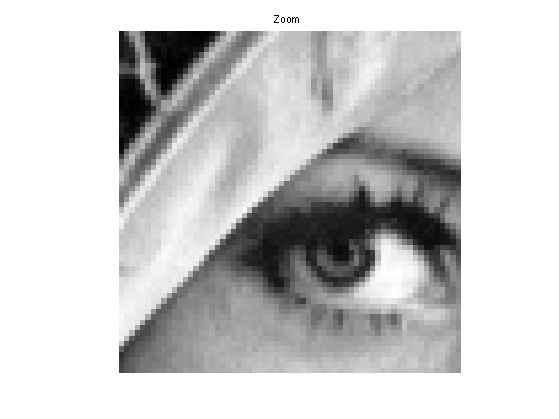An image is a 2D array, that can be modified as a matrix.

clf;
imageplot(-f, '-f', 1,2,1);
imageplot(f(n0:-1:1,:), 'Flipped', 1,2,2);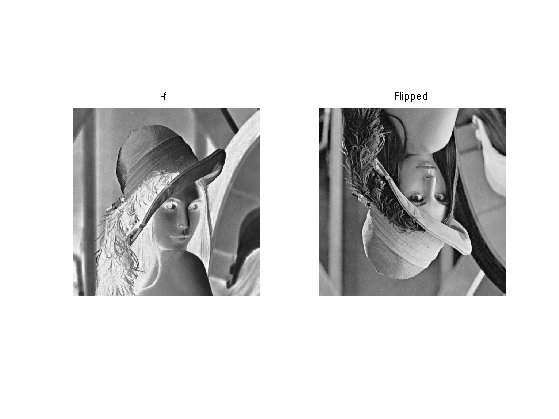Blurring is achieved by computing a convolution $$f \star h$$ with a kernel $$h$$.

Compute the low pass kernel.

k = 9; % size of the kernel
h = ones(k,k);
h = h/sum(h(:)); % normalize


Compute the convolution $$f \star h$$.

fh = perform_convolution(f,h);


Display.

clf;
imageplot(fh, 'Blurred image');## Fourier Transform

The Fourier orthonormal basis is defined as $\psi_m(k) = \frac{1}{\sqrt{N}}e^{\frac{2i\pi}{N_0} \dotp{m}{k} }$ where $$0 \leq k_1,k_2 < N_0$$ are position indexes, and $$0 \leq m_1,m_2 < N_0$$ are frequency indexes.

The Fourier transform $$\hat f$$ is the projection of the image on this Fourier basis $\hat f(m) = \dotp{f}{\psi_m}.$

The Fourier transform is computed in $$O(N \log(N))$$ operation using the FFT algorithm (Fast Fourier Transform). Note the normalization by $$\sqrt{N}=N_0$$ to make the transform orthonormal.

F = fft2(f) / n0;


We check this conservation of the energy.

disp(strcat(['Energy of Image:   ' num2str(norm(f(:)))]));
disp(strcat(['Energy of Fourier: ' num2str(norm(F(:)))]));

Energy of Image:   255.9831
Energy of Fourier: 255.9831


Compute the logarithm of the Fourier magnitude $$\log(\abs{\hat f(m)} + \epsilon)$$, for some small $$\epsilon$$.

L = fftshift(log( abs(F)+1e-1 ));


Display. Note that we use the function fftshift is useful to put the 0 low frequency in the middle.

clf;
imageplot(L, 'Log(Fourier transform)');## Linear Fourier Approximation

An approximation is obtained by retaining a certain set of index $$I_M$$ $f_M = \sum_{ m \in I_M } \dotp{f}{\psi_m} \psi_m.$

Linear approximation is obtained by retaining a fixed set $$I_M$$ of $$M = \abs{I_M}$$ coefficients. The important point is that $$I_M$$ does not depend on the image $$f$$ to be approximated.

For the Fourier transform, a low pass linear approximation is obtained by keeping only the frequencies within a square. $I_M = \enscond{m=(m_1,m_2)}{ -q/2 \leq m_1,m_2 < q/2 }$ where $$q = \sqrt{M}$$.

This can be achieved by computing the Fourier transform, setting to zero the $$N-M$$ coefficients outside the square $$I_M$$ and then inverting the Fourier transform.

Number $$M$$ of kept coefficients.

M = n0^2/64;


Exercice 1: (check the solution) Perform the linear Fourier approximation with $$M$$ coefficients. Store the result in the variable fM.

exo1;Compare two 1D profile (lines of the image). This shows the strong ringing artifact of the linea approximation.

clf;
subplot(2,1,1);
plot(f(:,n0/2));
axis('tight'); title('f');
subplot(2,1,2);
plot(fM(:,n0/2));
axis('tight'); title('f_M');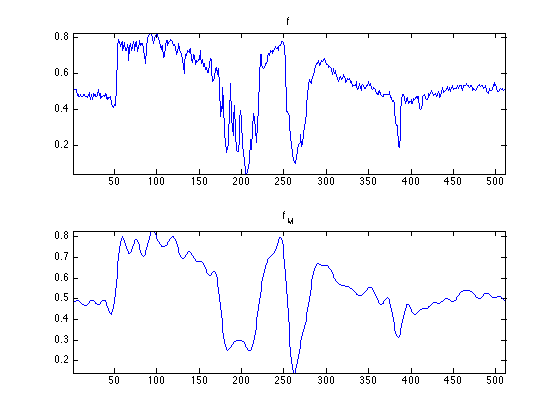## Non-linear Fourier Approximation

Non-linear approximation is obtained by keeping the $$M$$ largest coefficients. This is equivalently computed using a thresholding of the coefficients $I_M = \enscond{m}{ \abs{\dotp{f}{\psi_m}}>T }.$

Set a threshold $$T>0$$.

T = .2;


Compute the Fourier transform.

F = fft2(f) / n0;


Do the hard thresholding.

FT = F .* (abs(F)>T);


Display. Note that we use the function fftshift is useful to put the 0 low frequency in the middle.

clf;
L = fftshift(log( abs(FT)+1e-1 ));
imageplot(L, 'thresholded Log(Fourier transform)');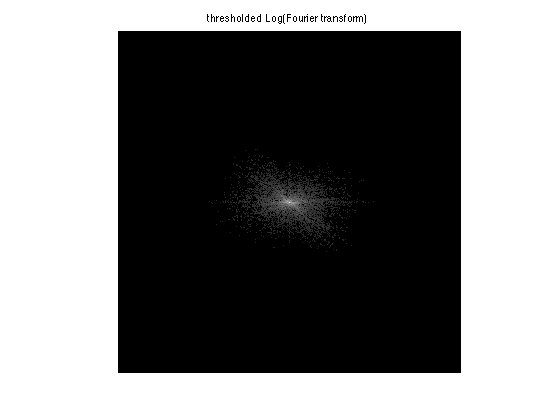Inverse Fourier transform to obtained $$f_M$$

fM = real( ifft2(FT)*n0 );


Display.

clf;
imageplot(clamp(fM), ['Non-linear, Fourier, SNR=' num2str(snr(f,fM), 4) 'dB']);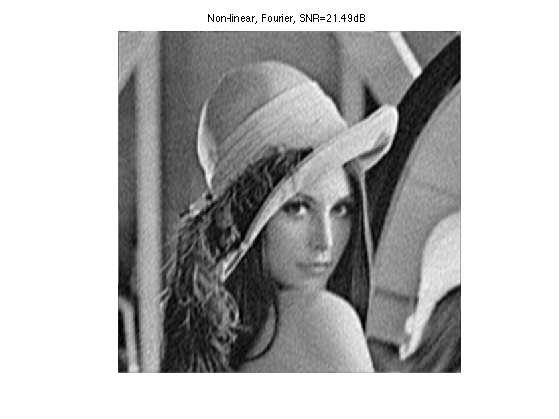Given a $$T$$, the number of coefficients is obtained by counting the non thresholded coefficients $$\abs{I_M}$$.

m = sum(FT(:)~=0);
disp(['M/N = 1/'  num2str(round(n0^2/m)) '.']);

M/N = 1/32.


Exercice 2: (check the solution) Compute the value of the threshold $$T$$ so that the number of coefficients is $$M$$. Display the corresponding approximation $$f_M$$.

exo2;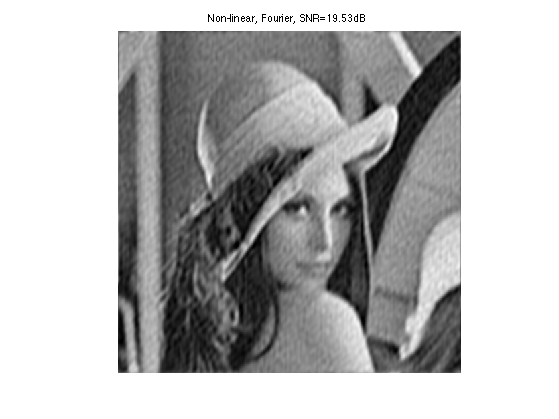## Wavelet Transform

A wavelet basis $$\Bb = \{ \psi_m \}_m$$ is obtained over the continuous domain by translating an dilating three mother wavelet functions $$\{\psi^V,\psi^H,\psi^D\}$$.

Each wavelet atom is defined as $\psi_m(x) = \psi_{j,n}^k(x) = \frac{1}{2^j}\psi^k\pa{ \frac{x-2^j n}{2^j} }.$ The scale (size of the support) is $$2^j$$ and the position is $$2^j(n_1,n_2)$$. The index is $$m=(k,j,n)$$ for \{ j \leq 0 \}.

The wavelet transform computes all the inner products $$\{ \dotp{f}{\psi_{j,n}^k} \}_{k,j,n}$$.

Set the minimum scale for the transform to be 0.

Jmin = 0;


Perform the wavelet transform, fw stores all the wavelet coefficients.

fw = perform_wavelet_transf(f,Jmin,+1);


Display the transformed coefficients.

clf;
plot_wavelet(fw);## Wavelet Approximation

Linear wavelet approximation with $$M=2^{-j_0}$$ coefficients is obtained by keeping only the coarse scale (large support) wavelets: $I_M = \enscond{(k,j,n)}{ j \geq j_0 }.$

It corresponds to setting to zero all the coefficients excepted those that are on the upper left corner of fw.

Exercice 3: (check the solution) Perform linear approximation with $$M$$ wavelet coefficients.

exo3;A non-linear approximation is obtained by keeping the $$M$$ largest wavelet coefficients.

As already said, this is equivalently computed by a non-linear hard thresholding.

Select a threshold.

T = .2;


Perform hard thresholding.

fwT = fw .* (abs(fw)>T);


Display the thresholded coefficients.

clf;
subplot(1,2,1);
plot_wavelet(fw);
title('Original coefficients');
subplot(1,2,2);
plot_wavelet(fwT);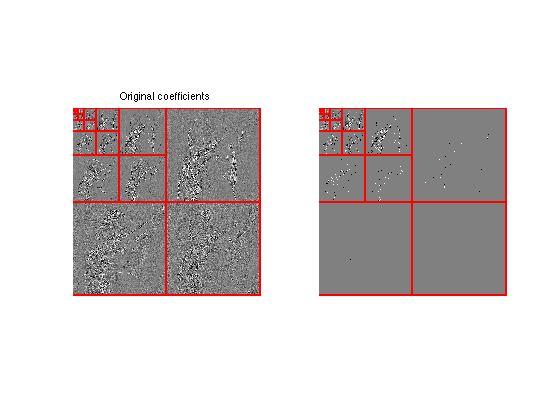Perform reconstruction.

fM = perform_wavelet_transf(fwT,Jmin,-1);


Display approximation.

clf;
imageplot(clamp(fM), strcat(['Approximation, SNR=' num2str(snr(f,fM),3) 'dB']));Exercice 4: (check the solution) Perform non-linear approximation with $$M$$ wavelet coefficients by chosing the correct value for $$T$$. Store the result in the variable fM.

exo4;Compare two 1D profile (lines of the image). Note how the ringing artifacts are reduced with respec to the Fourier approximation.

clf;
subplot(2,1,1);
plot(f(:,n0/2));
axis('tight'); title('f');
subplot(2,1,2);
plot(fM(:,n0/2));
axis('tight'); title('f_M');